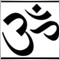# this works, this idea reasonable? help is very much appreciated117

thi high of the last 2 H1 bars-the low of the last two H1 bars could be....

double HighMinusLow_H2=  MathMax(iHigh(NULL,PERIOD_ H1,  1),  iHigh(NULL,  PERIOD_H1, 2))-  MathMin(iLow(NULL, PERIOD_H1, 1), iLow(NULL, PERIOD_H1, 2));

Now i want to take the current bar of High and then subtract the low of last two  hour. how do i do that.  help appreciated5300

Current_Bar High=

```    iHigh(Symbol(),PERIOD_H1,0);
/*
Symbol because I don't like Null.
Period_H1 for Hourly.
Zero for current bar.
*/```

If you meant something else than explain.thi high of the last 2 H1 bars-the low of the last two H1 bars could be....

double HighMinusLow_H2=  MathMax(iHigh(NULL,PERIOD_ H1,  1),  iHigh(NULL,  PERIOD_H1, 2))-  MathMin(iLow(NULL, PERIOD_H1, 1), iLow(NULL, PERIOD_H1, 2));

Now i want to take the current bar of High and then subtract the low of last two  hour. how do i do that.  help appreciated25313

how do i do that. help appreciated
You should THINK before posting (and don't double post):
 Your code: high of the last 2 H1 bars-the low of the last two H1 ```double HighLastTwo = MathMax(iHigh(NULL, PERIOD_ H1,1), iHigh(NULL, PERIOD_H1, 2))         LowLastTwo = MathMin( iLow(NULL, PERIOD_H1, 1),  iLow(NULL, PERIOD_H1, 2)),        HighMinusLow_H2= HighLastTwo = LowLastTwo;``` Now i want to take the current bar of High and then subtract the low of last two  hour ```double HighCurrent = High, // or iHigh(NULL, PERIOD_H1, 0),         LowLastTwo = MathMin( iLow(NULL, PERIOD_H1, 1),  iLow(NULL, PERIOD_H1, 2)),        CurHighMinusLow_H2= HighCurrent = LowLastTwo;```
Was that so hard?